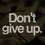# Cauchy-Schwarz Training

The biggest real value of the expression $\cos{x} \cdot ( 2\sin{x} + 4\cos{x})$ can be written as $a+ \sqrt{b}$, where $a$ and $b$ are positive square-free integers.

The smallest real value of the expression $ay^2 + \dfrac{b}{y^2}$ can be written as $c \cdot \sqrt{d}$, where $c$ and $d$ are positive square-free integers. Restrict $x$ and $y$ to the real numbers.

1. Show that $ab = bc = d$.

2. Find the monic polynomial $P(x)$ of fourth degree that has $a,b,c,d$ as its roots.

3. Find $P(x)$ 's smallest real value.Note by Guilherme Dela Corte
5 years, 8 months ago

This discussion board is a place to discuss our Daily Challenges and the math and science related to those challenges. Explanations are more than just a solution — they should explain the steps and thinking strategies that you used to obtain the solution. Comments should further the discussion of math and science.

When posting on Brilliant:

• Use the emojis to react to an explanation, whether you're congratulating a job well done , or just really confused .
• Ask specific questions about the challenge or the steps in somebody's explanation. Well-posed questions can add a lot to the discussion, but posting "I don't understand!" doesn't help anyone.
• Try to contribute something new to the discussion, whether it is an extension, generalization or other idea related to the challenge.

MarkdownAppears as
*italics* or _italics_ italics
**bold** or __bold__ bold
- bulleted- list
• bulleted
• list
1. numbered2. list
1. numbered
2. list
Note: you must add a full line of space before and after lists for them to show up correctly
paragraph 1paragraph 2

paragraph 1

paragraph 2

[example link](https://brilliant.org)example link
> This is a quote
This is a quote
    # I indented these lines
# 4 spaces, and now they show
# up as a code block.

print "hello world"
# I indented these lines
# 4 spaces, and now they show
# up as a code block.

print "hello world"
MathAppears as
Remember to wrap math in $$ ... $$ or $ ... $ to ensure proper formatting.
2 \times 3 $2 \times 3$
2^{34} $2^{34}$
a_{i-1} $a_{i-1}$
\frac{2}{3} $\frac{2}{3}$
\sqrt{2} $\sqrt{2}$
\sum_{i=1}^3 $\sum_{i=1}^3$
\sin \theta $\sin \theta$
\boxed{123} $\boxed{123}$

Sort by:

Write a comment or ask a question...Find K so that U and V are orthogonal where U=(2,3K,-4,1,-5) and V=(6,-1,3,7,2K)

- 5 years, 2 months ago

Waht's the question which has no answer ?

- 5 years, 8 months ago

All questions have answers! However, #1 is a proof, and thus has not a closed-form value to submit.

- 5 years, 8 months ago

Can anyone tell me about Cauchy-Schwarz inequality? I know the AM-GM inequality but not this. Please illustrate some simple examples too (I am new to this inequality.).

- 5 years, 8 months ago

If you type in Cauchy-Schwarz in the search box there would be a featured community post on Cauchy-Schwarz. There's a lot of information there.

- 5 years, 8 months ago

The first expression can be reduced to $\sin 2x+2\cos 2x+2$.

$(\sin 2x+2\cos 2x)^{2} \leq (\sin^{2} 2x+ \cos^{2} 2x)(1+4)=5$ hence the maximum value is $2+\sqrt {5}$. Equality can occur when $\tan x=0.5$.

Now $c, d$ can be found by AM-GM. However, since Cauchy-Schwarz needs to be used, we do it in a slightly different way.

$(2y^{2}+\frac {5}{y^{2}})(\frac {5}{y^{2}}+2y^{2}) \geq (\sqrt {10}+\sqrt {10})^{2}=40$ hence $2y^{2}+\frac {5}{y^{2}} \geq \sqrt {40}=2\sqrt {10}$.

Hence $a=2, b=5, c=2, d=10$.

1. Clearly $2×5=5×2=10$.
2. $P (x)=(x-2)^{2}(x-5)(x-10)$
3. Using Cauchy-Schwarz rather than calculus is difficult, but here's a solution:

By Cauchy-Schwarz, $(a^{2}+b^{2})(b^{2}+a^{2}) \geq (ab+ba)^{2}$ hence $a^{2}+b^{2} \geq 2ab$ for reals $a, b$.

Thus for all positive reals $a, b, c, d$, $a^{4}+b^{4}+c^{4}+d^{4} \geq 2a^{2} b^{2}+2c^{2} d^{2} \geq 4abcd$ by applying the above twice. We will use this fact.

Clearly the polynomial can have negative values. Just fit in x=7.

Now the only way to get negative values is to have $(x-5)(x-10)$ negative, or $5 < x <10$. Hence $x-2, x-5, 10-x$ are positive. So an easier problem would be to find the maximum of $(x-2)(x-2)(x-5)(10-x)$.

Now, using the fact above, $r(x-2)r (x-2)(1-2r)(x-5)(10-x) \leq (\frac {r(x-2)+r (x-2)+(1-2r)(x-5)+10-x}{4})^{4}=(\frac {6r+5}{4})^{4}$. So the maximum, if equality can occur, is $\frac {(6r+5)^{4}}{256r^{2}(1-2r)}$.

In particular, when $r=\frac {21-\sqrt {321}}{12}$, we get the maximum as that is the only case equality can occur. (Remember that the corresponding terms in Cauchy-Schwarz have to be related by a common ratio for equality.) The minimum is the negative of the maximum and is approximately -223. Equality can occur at $x=\frac {64-4\sqrt {321}}{17-\sqrt {321}}$.

- 5 years, 8 months ago

a) Why is the "maximum" $\dfrac{(6r+5)^4}{256r^2 (1-2r)}$ ? How did attain it?

b) Why is $r = \dfrac{21 - \sqrt{321}}{12}$ ?

- 5 years, 8 months ago

a) it is by rearranging the terms in the inequality. b) By Cauchy, equality can occur only when r(x-2)=(1-2r)(x-5)=10-x. Solving gives r.

- 5 years, 8 months ago

Challenge: Can the third question be answered by Cauchy-Schwarz?

- 5 years, 8 months ago